# Selina Solutions Concise Mathematics Class 6 Chapter 12 Proportion

Selina Solutions Concise Mathematics Class 6 Chapter 12 provides the basic idea about the concepts, which are vital from the exam point of view. In-depth practice of textbook questions, help students to gain interest in Mathematics, along with improving their analytical thinking. The various examples present in the textbook help students to understand the method of solving the difficult questions effortlessly. Download the free PDF of Selina Solutions Concise Mathematics Class 6 Chapter 12 Proportion, from the links given below

Class 12 has problems on the main concept Proportions. If the two ratios are equal, then they are said to be in Proportion. Solutions provided in simple language help students to gain a better hold on the fundamental concepts. This also helps them to perform well in the annual examination.

## Selina Solutions Concise Mathematics Class 6 Chapter 12: Proportion Download PDF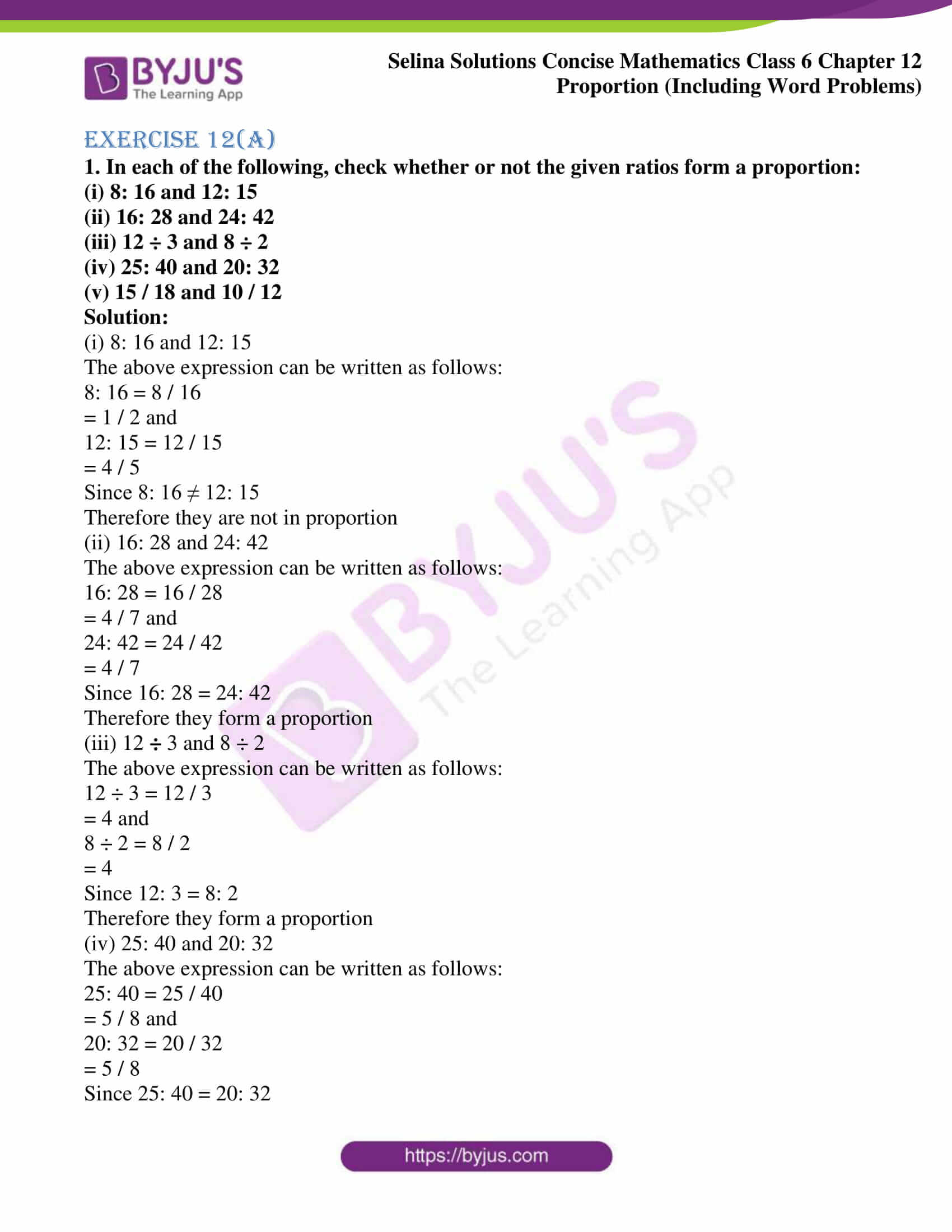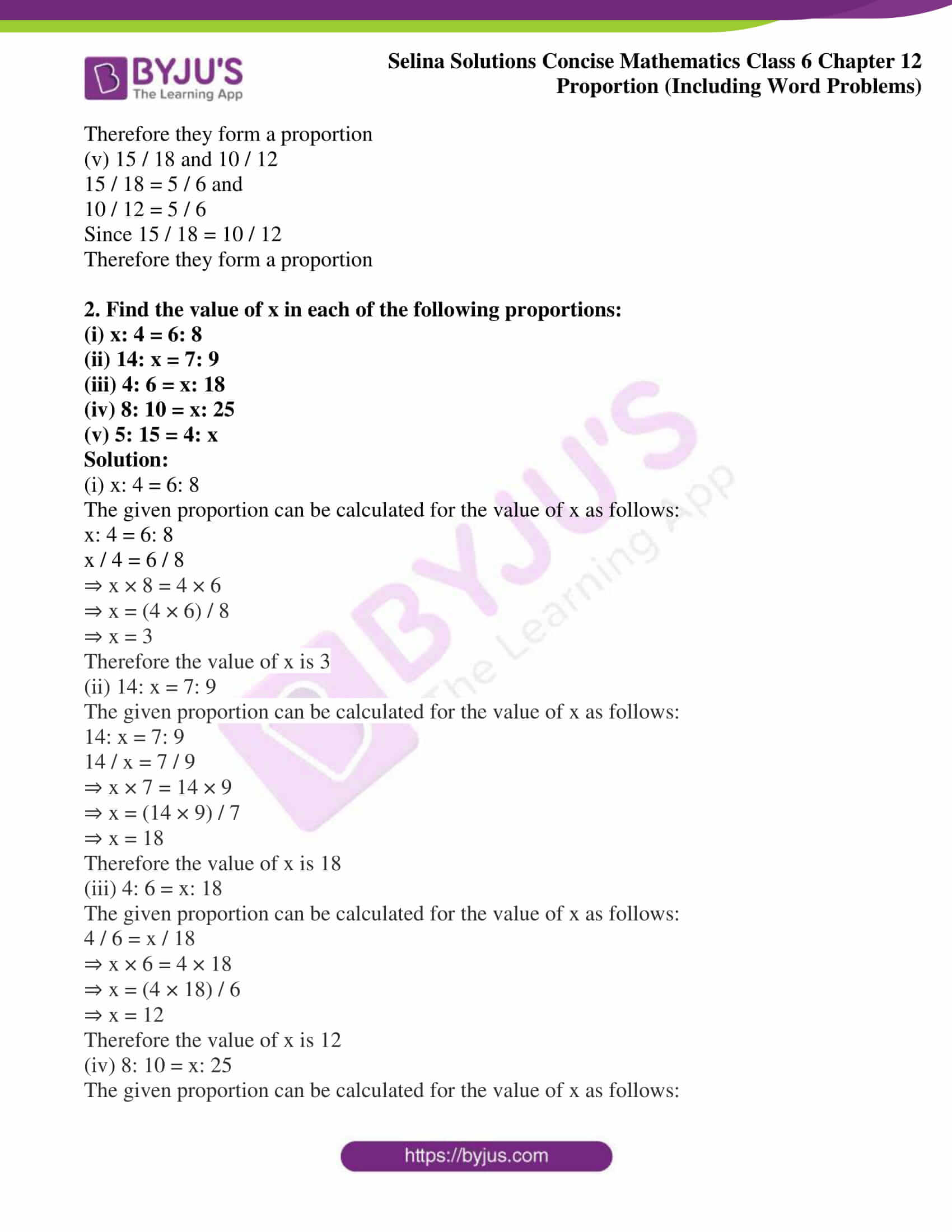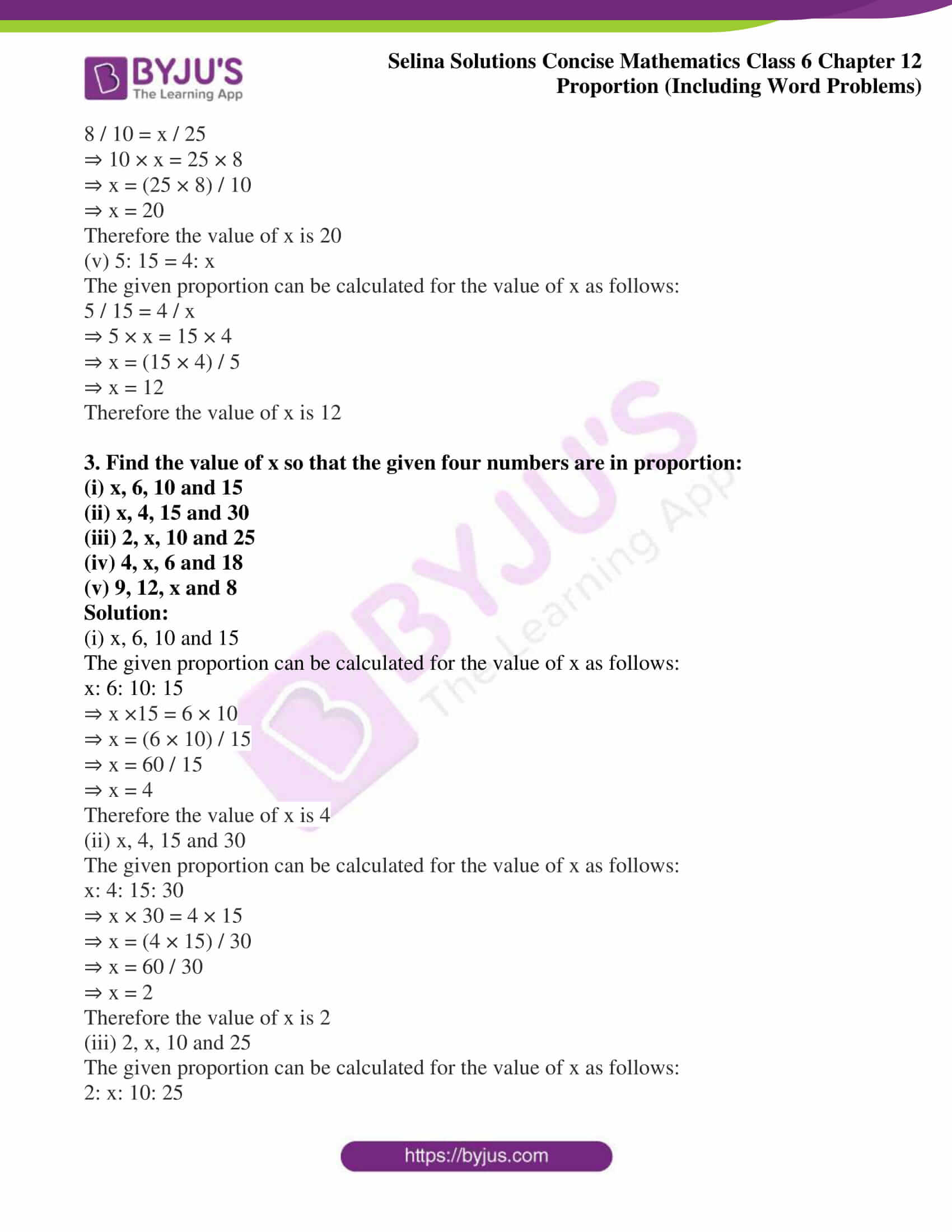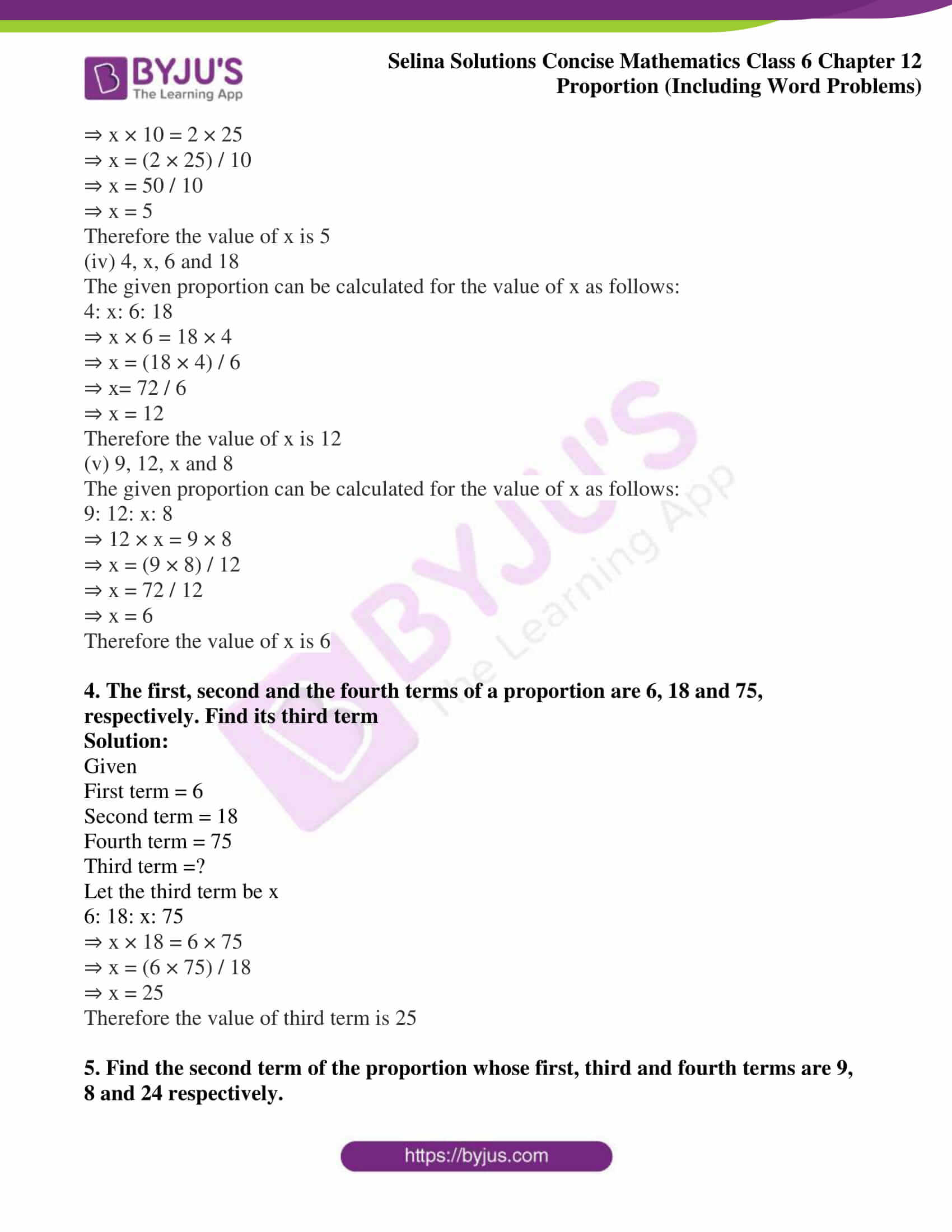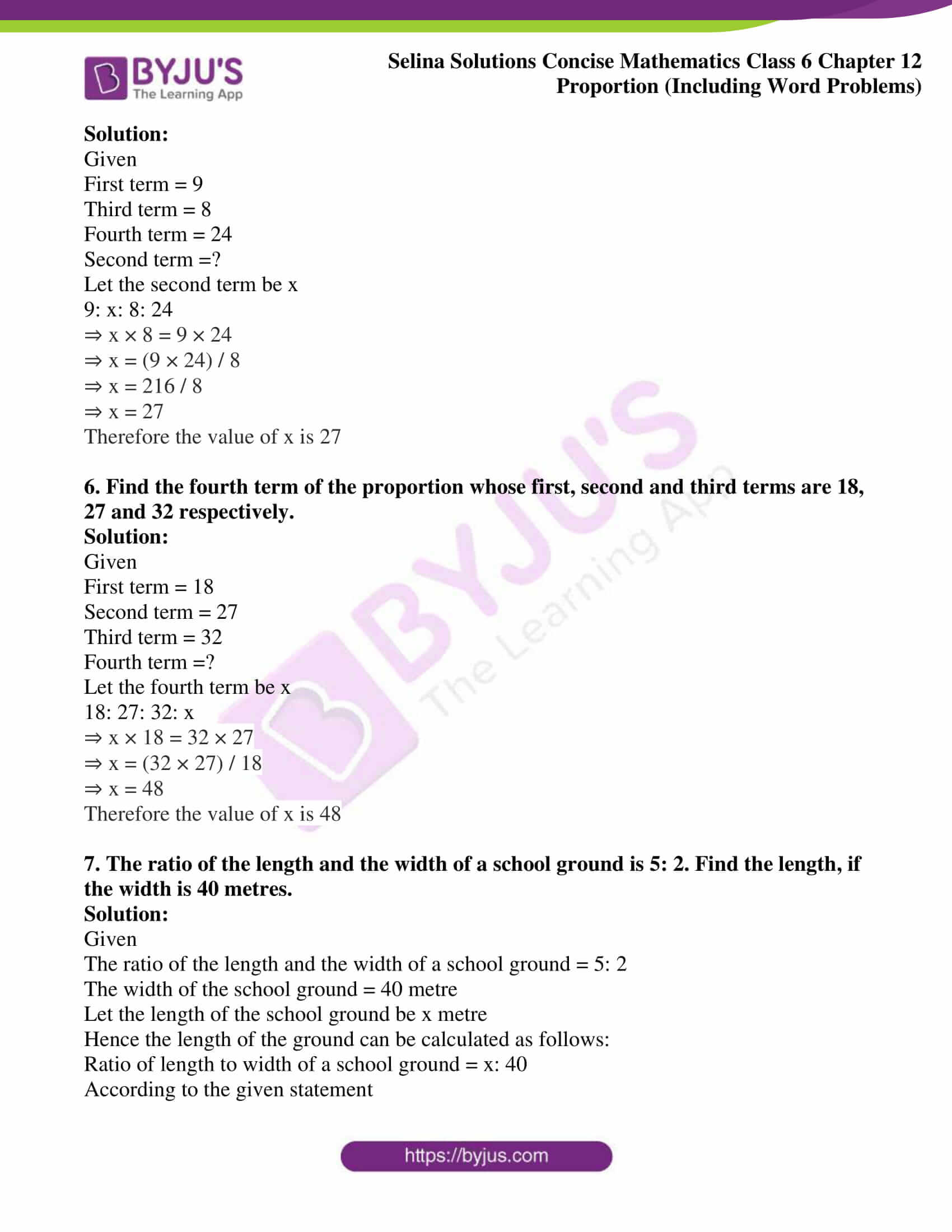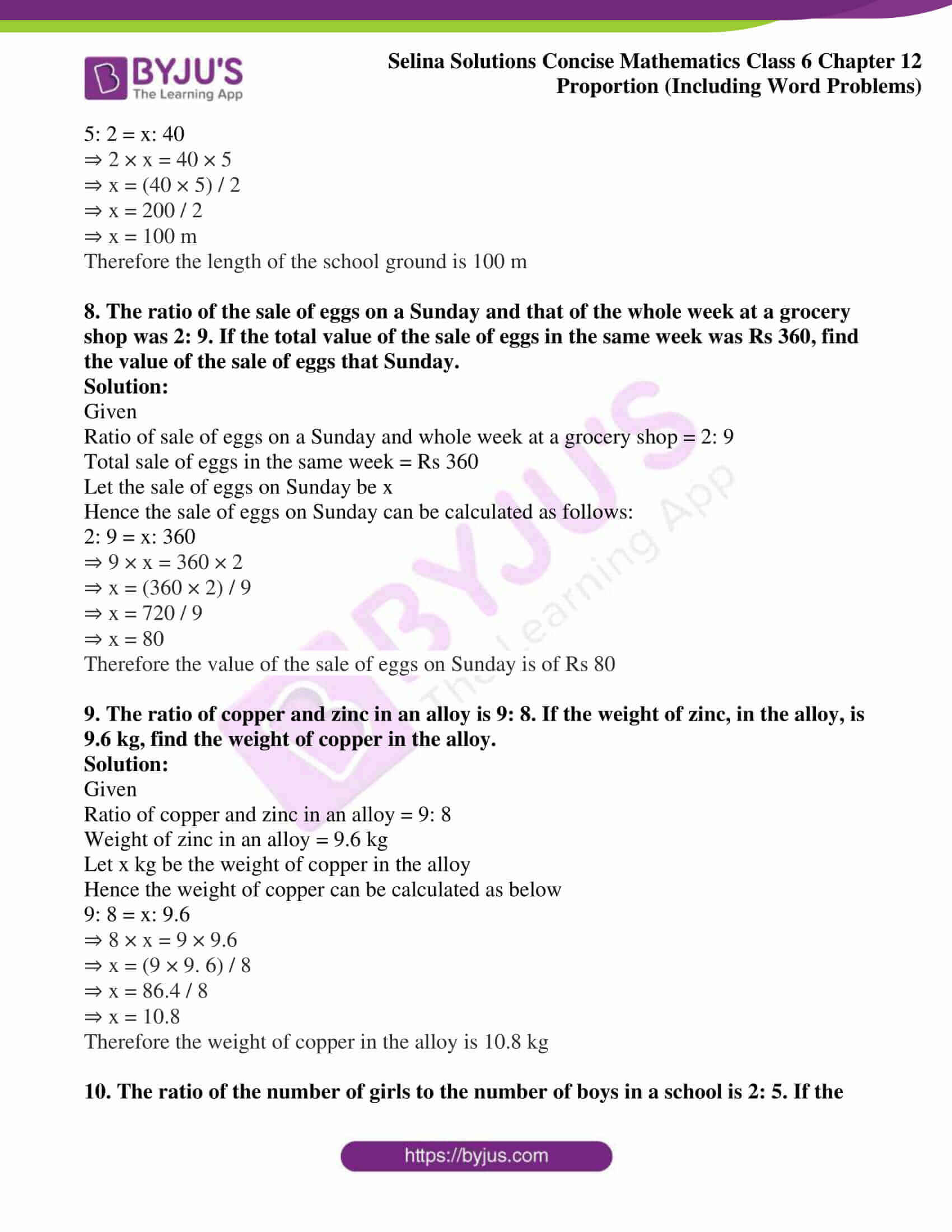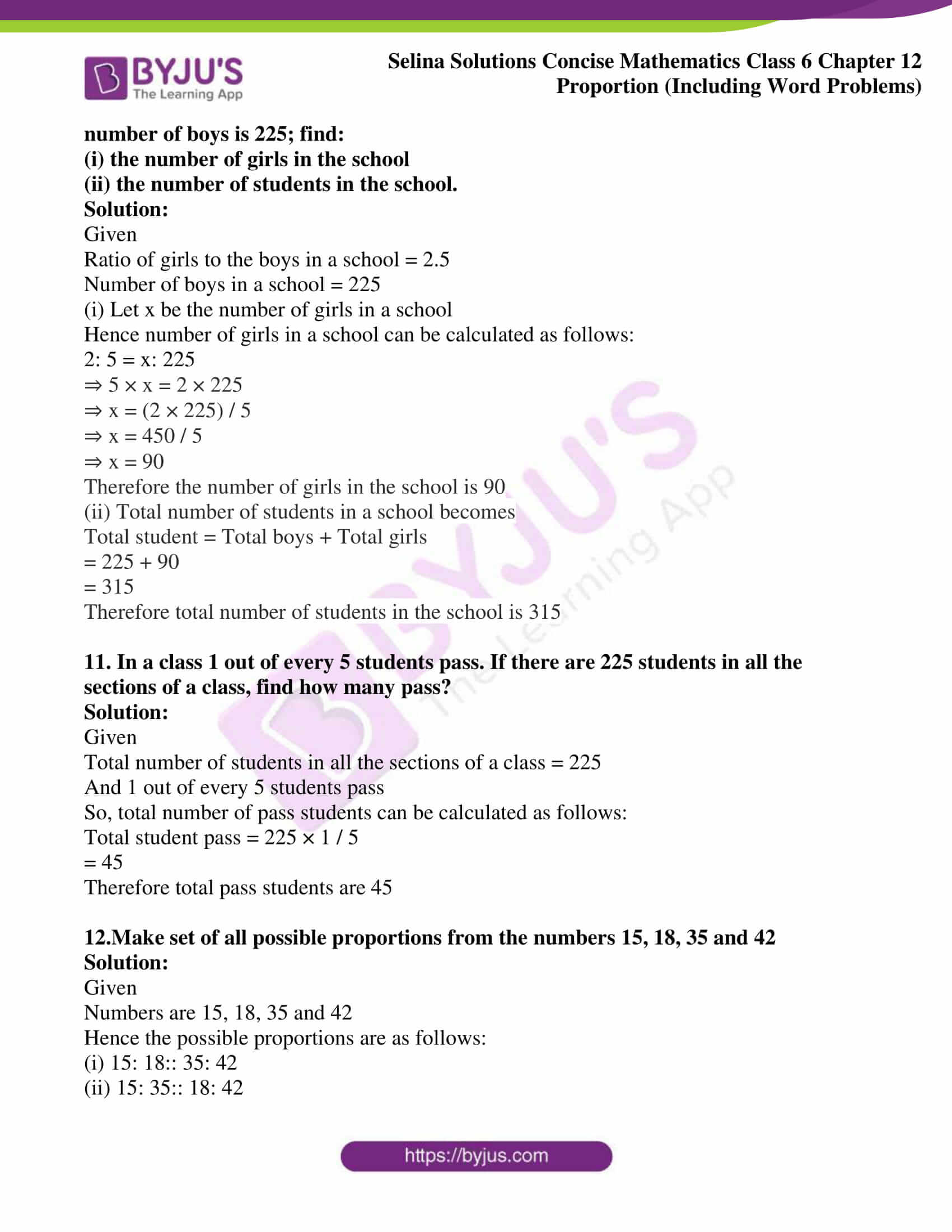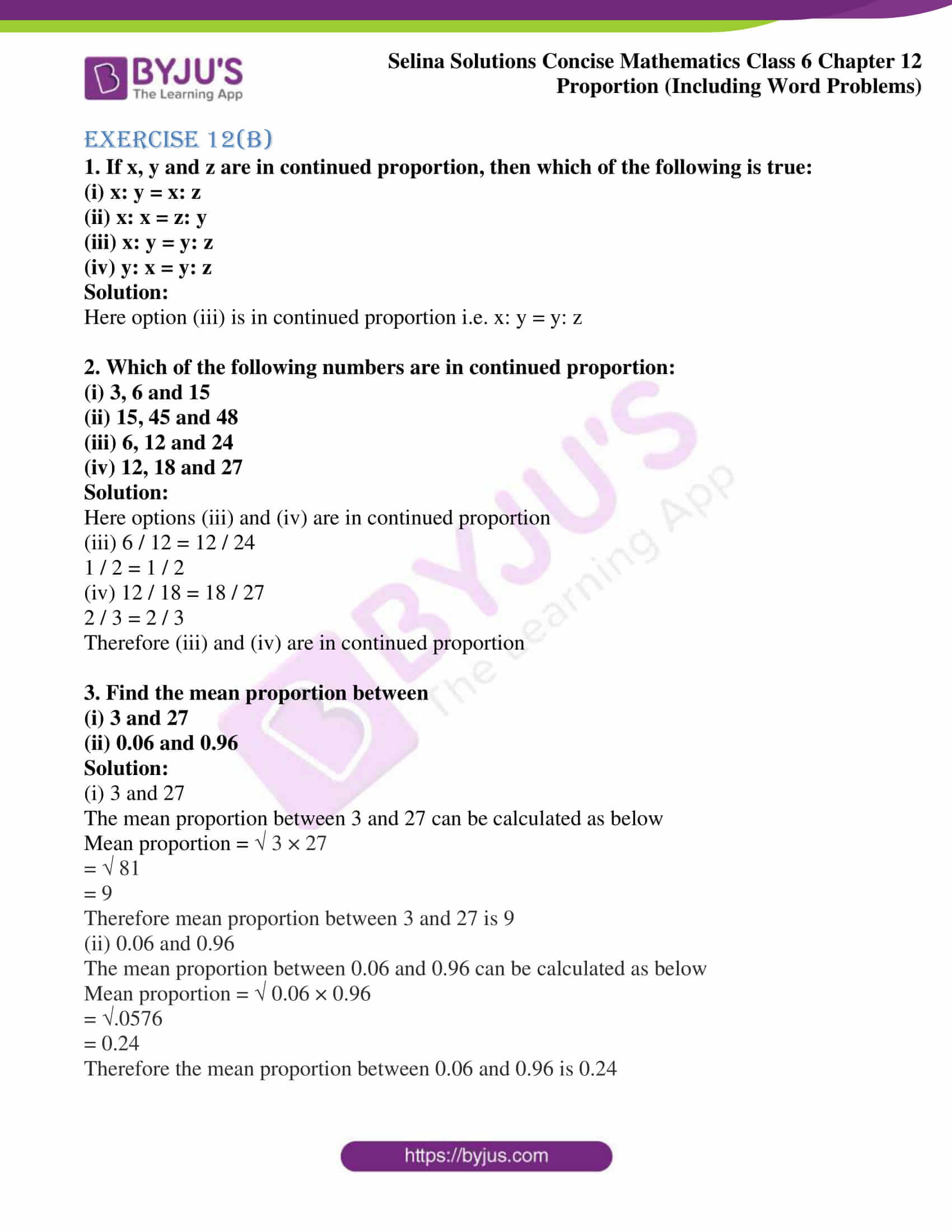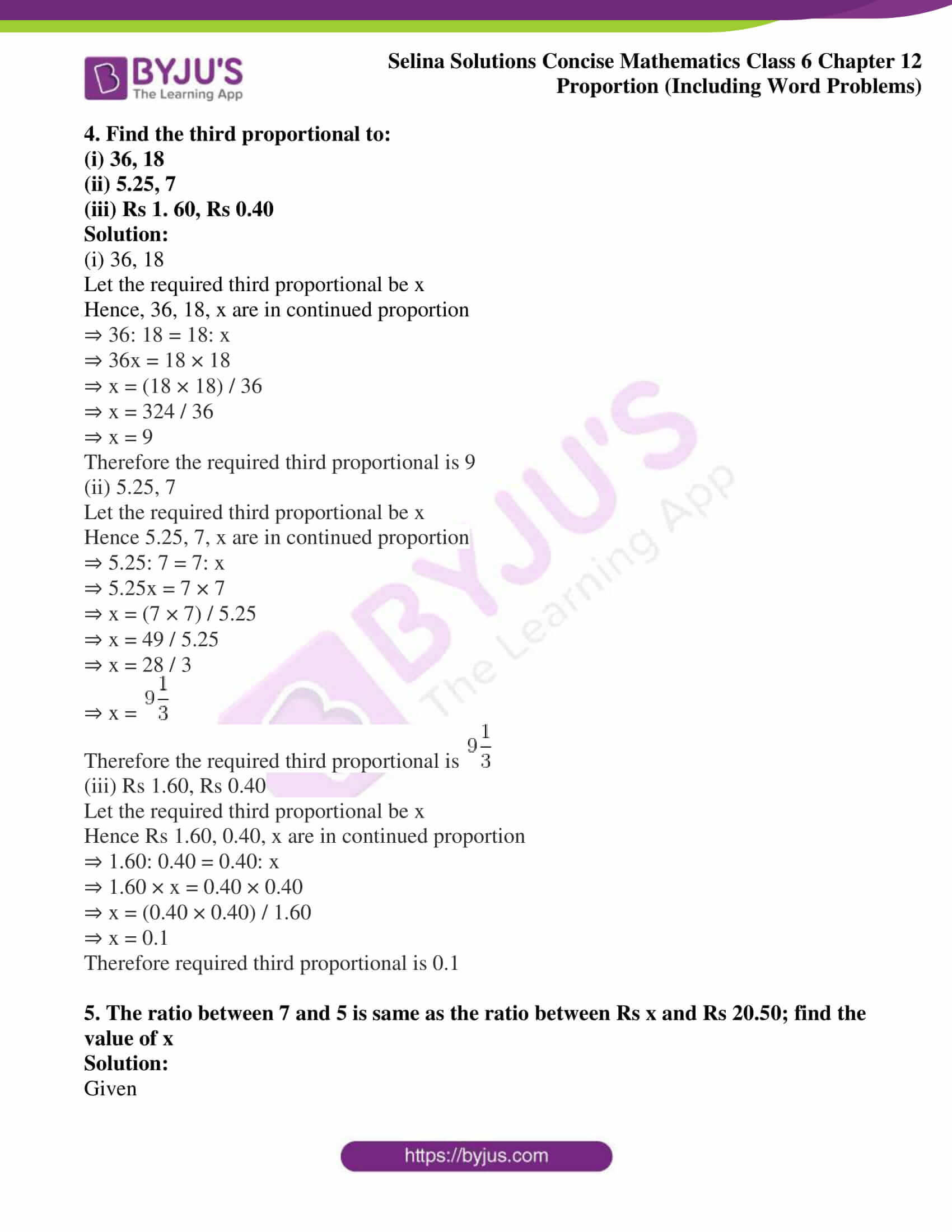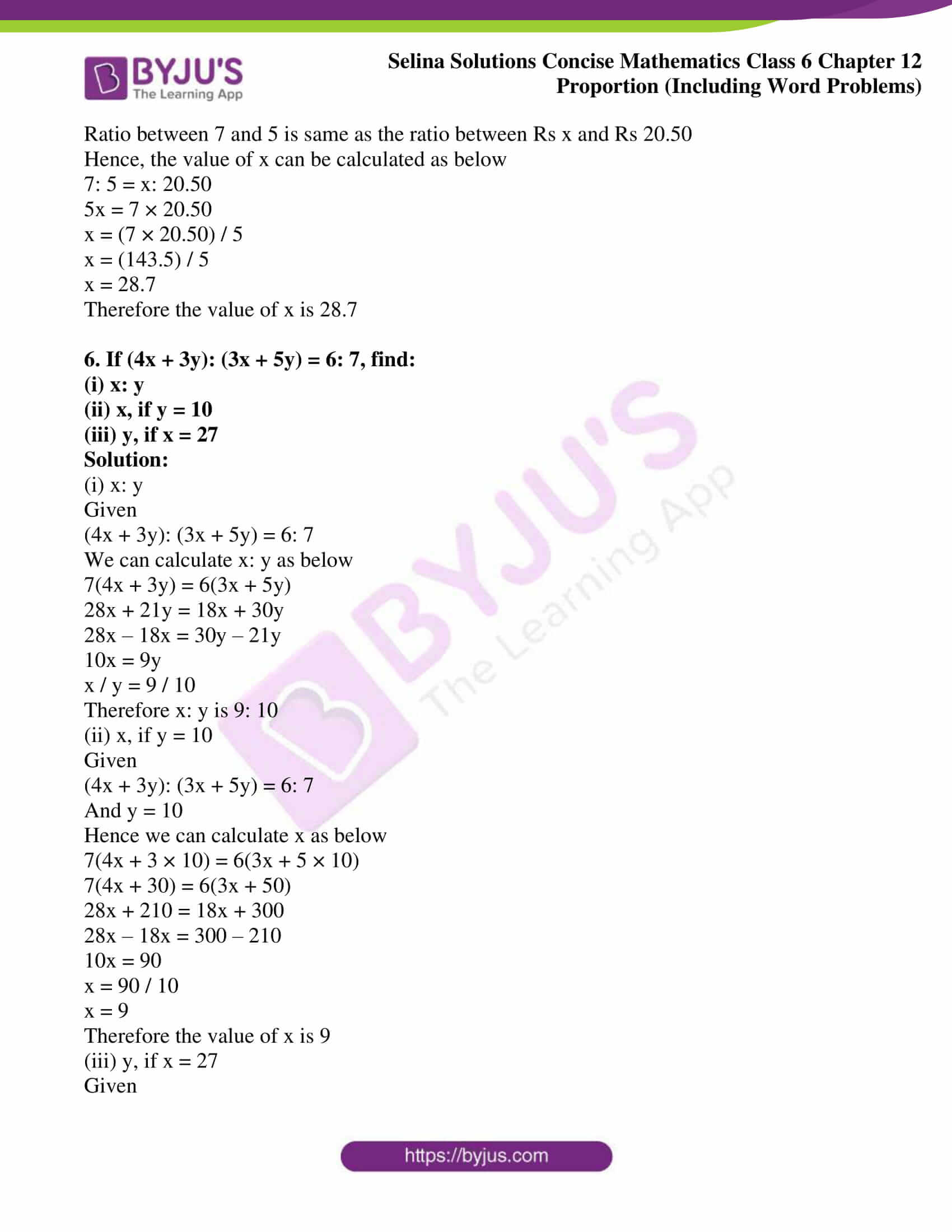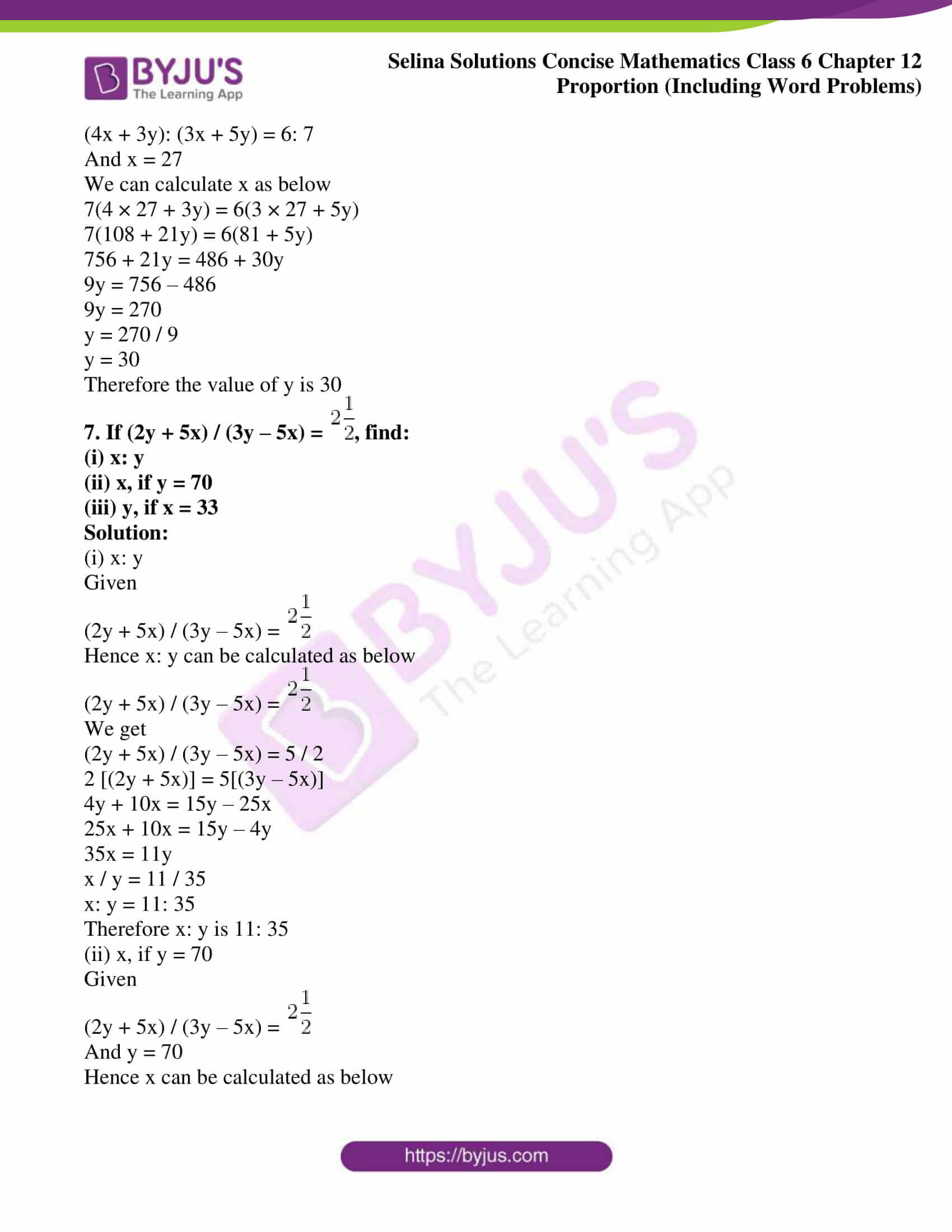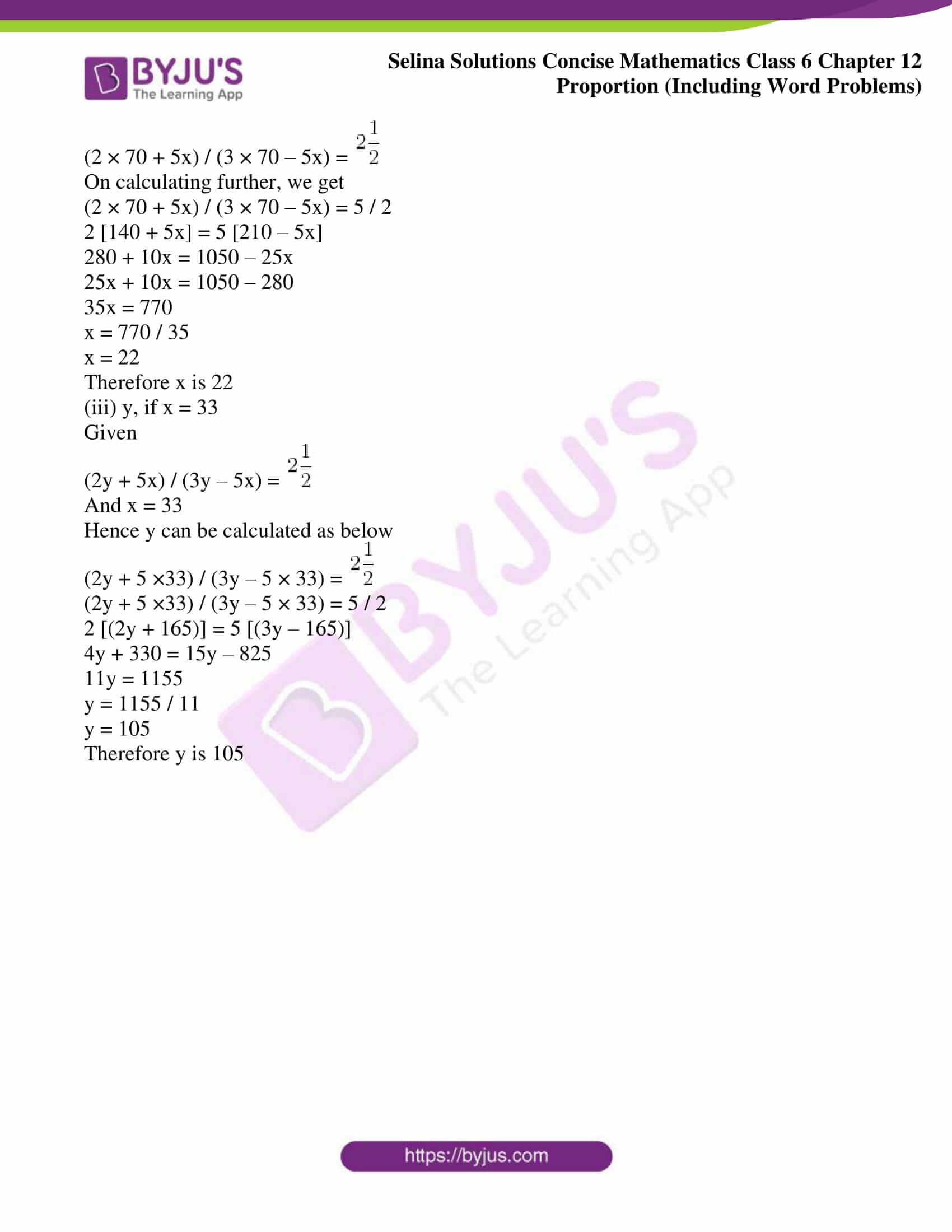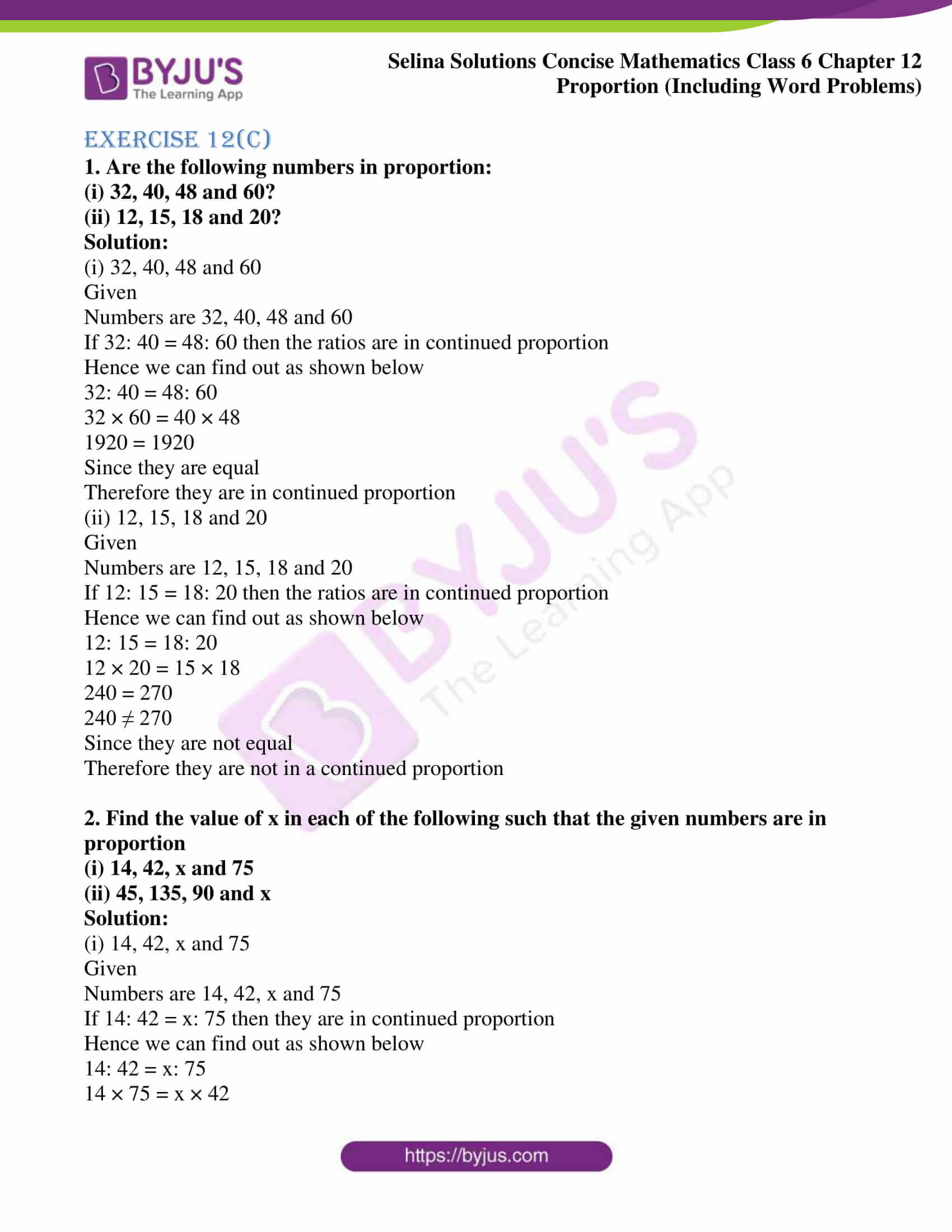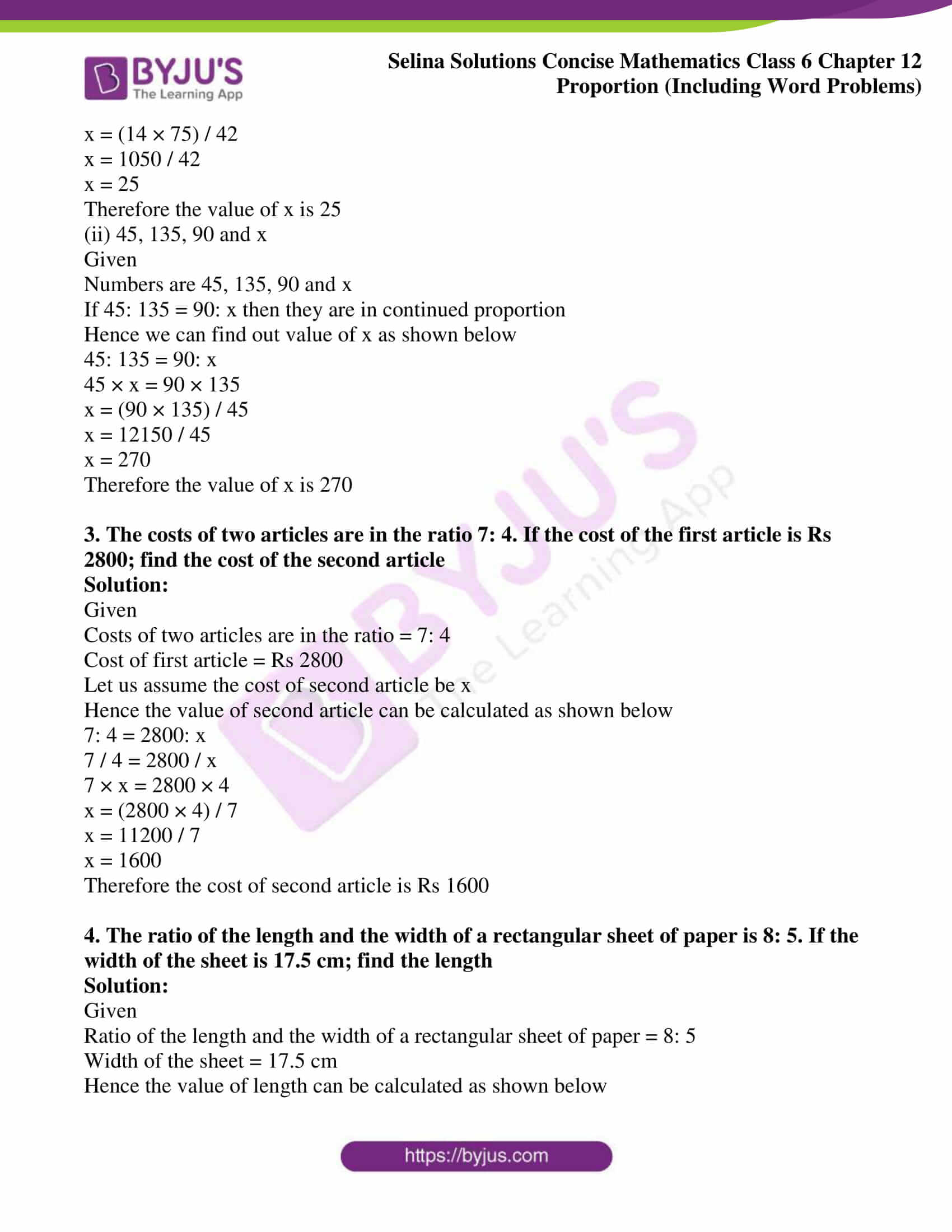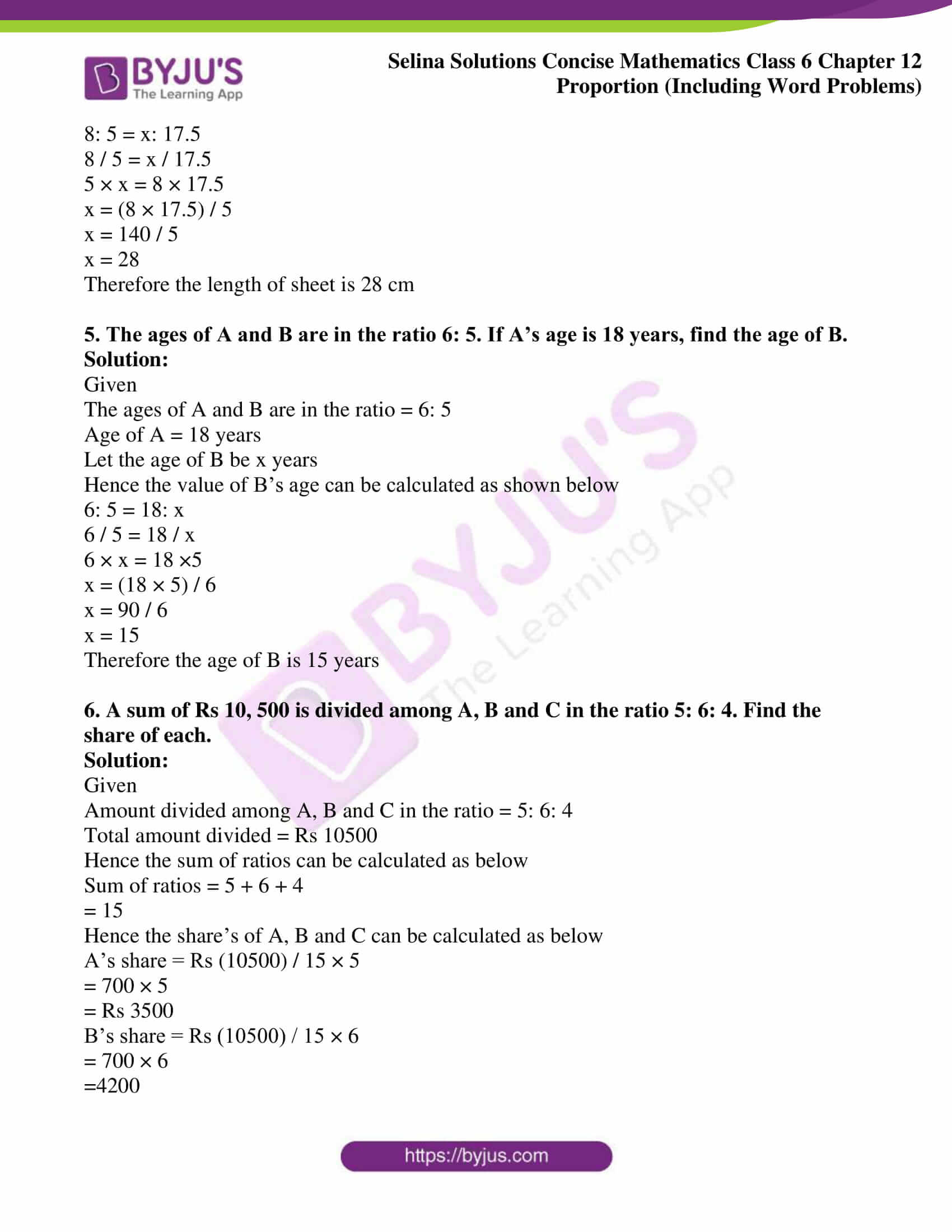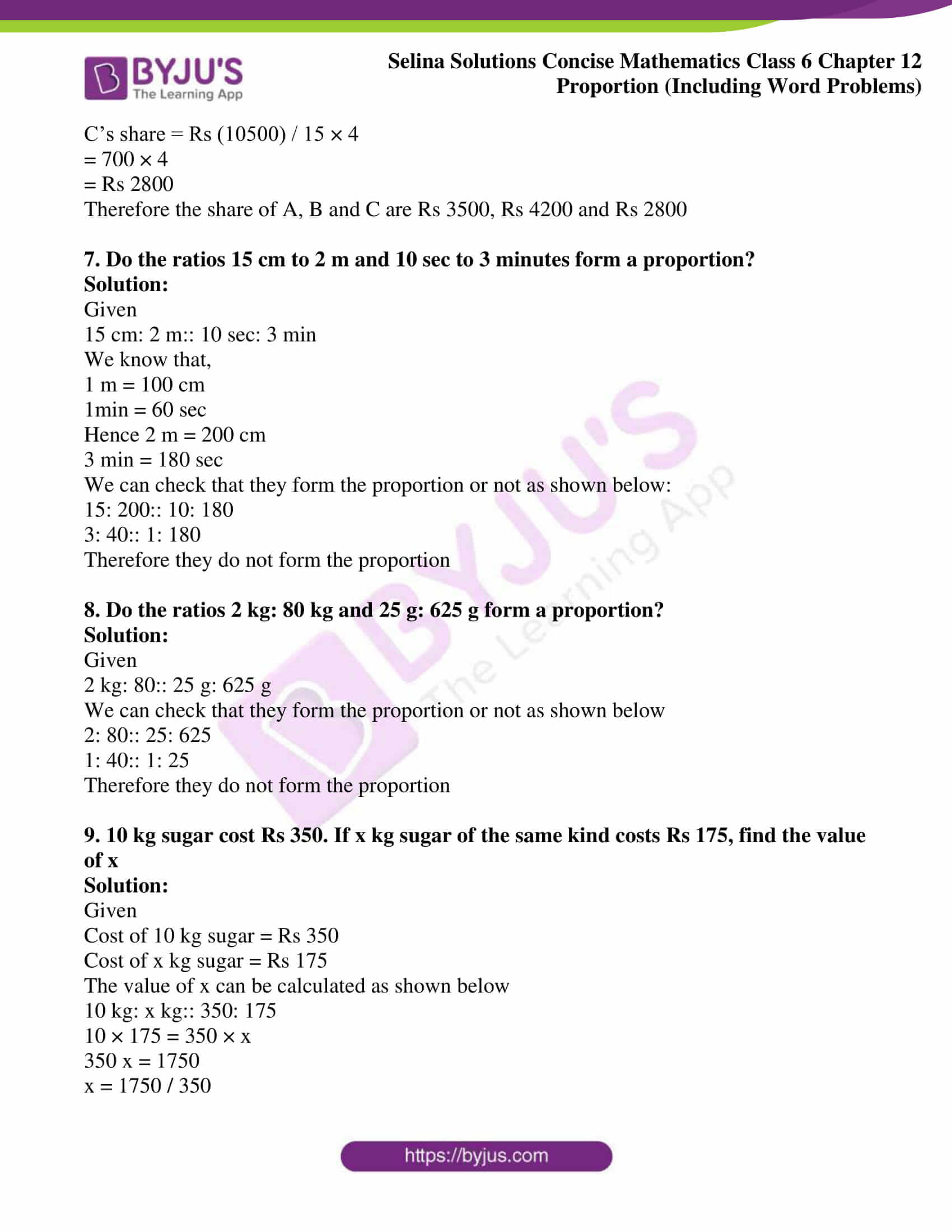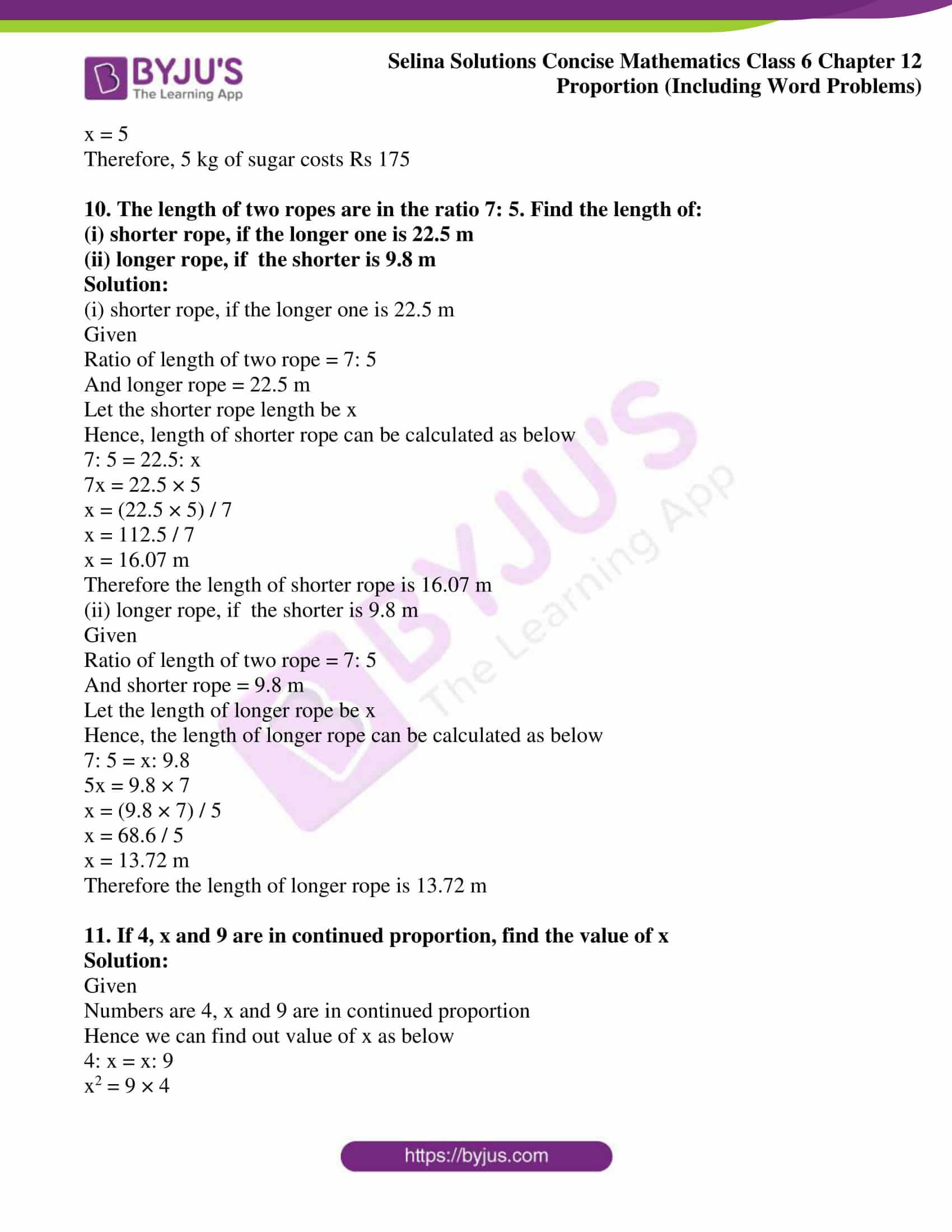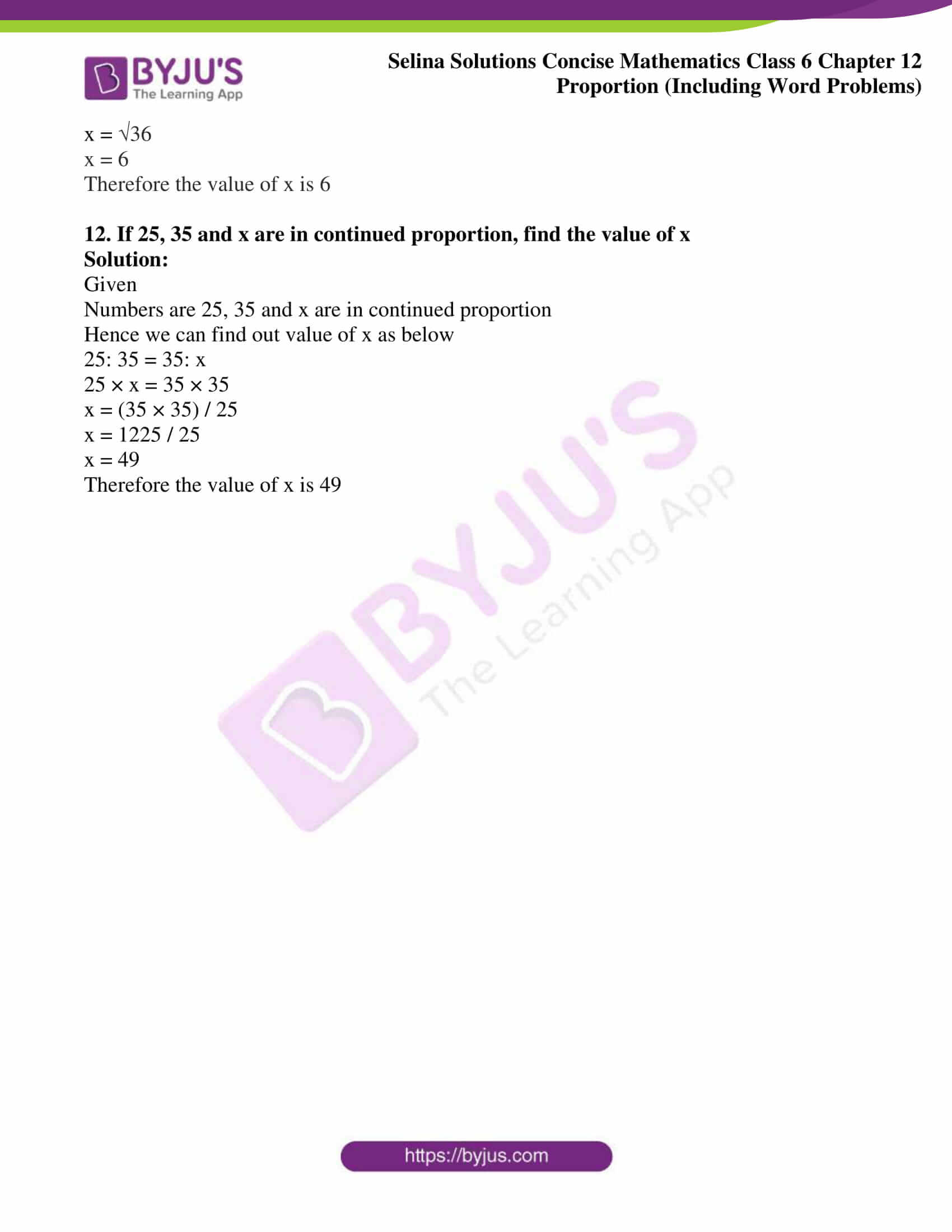## Access Selina Solutions Concise Mathematics Class 6 Chapter 12: Proportion

Exercise 12(A)

1. In each of the following, check whether or not the given ratios form a proportion:

(i) 8: 16 and 12: 15

(ii) 16: 28 and 24: 42

(iii) 12 ÷ 3 and 8 ÷ 2

(iv) 25: 40 and 20: 32

(v) 15 / 18 and 10 / 12

Solution:

(i) 8: 16 and 12: 15

The above expression can be written as follows:

8: 16 = 8 / 16

= 1 / 2 and

12: 15 = 12 / 15

= 4 / 5

Since 8: 16 ≠ 12: 15

Therefore they are not in proportion

(ii) 16: 28 and 24: 42

The above expression can be written as follows:

16: 28 = 16 / 28

= 4 / 7 and

24: 42 = 24 / 42

= 4 / 7

Since 16: 28 = 24: 42

Therefore they form a proportion

(iii) 12 ÷ 3 and 8 ÷ 2

The above expression can be written as follows:

12 ÷ 3 = 12 / 3

= 4 and

8 ÷ 2 = 8 / 2

= 4

Since 12: 3 = 8: 2

Therefore they form a proportion

(iv) 25: 40 and 20: 32

The above expression can be written as follows:

25: 40 = 25 / 40

= 5 / 8 and

20: 32 = 20 / 32

= 5 / 8

Since 25: 40 = 20: 32

Therefore they form a proportion

(v) 15 / 18 and 10 / 12

15 / 18 = 5 / 6 and

10 / 12 = 5 / 6

Since 15 / 18 = 10 / 12

Therefore they form a proportion

2. Find the value of x in each of the following proportions:

(i) x: 4 = 6: 8

(ii) 14: x = 7: 9

(iii) 4: 6 = x: 18

(iv) 8: 10 = x: 25

(v) 5: 15 = 4: x

Solution:

(i) x: 4 = 6: 8

The given proportion can be calculated for the value of x as follows:

x: 4 = 6: 8

x / 4 = 6 / 8

⇒ x × 8 = 4 × 6

⇒ x = (4 × 6) / 8

⇒ x = 3

Therefore the value of x is 3

(ii) 14: x = 7: 9

The given proportion can be calculated for the value of x as follows:

14: x = 7: 9

14 / x = 7 / 9

⇒ x × 7 = 14 × 9

⇒ x = (14 × 9) / 7

⇒ x = 18

Therefore the value of x is 18

(iii) 4: 6 = x: 18

The given proportion can be calculated for the value of x as follows:

4 / 6 = x / 18

⇒ x × 6 = 4 × 18

⇒ x = (4 × 18) / 6

⇒ x = 12

Therefore the value of x is 12

(iv) 8: 10 = x: 25

The given proportion can be calculated for the value of x as follows:

8 / 10 = x / 25

⇒ 10 × x = 25 × 8

⇒ x = (25 × 8) / 10

⇒ x = 20

Therefore the value of x is 20

(v) 5: 15 = 4: x

The given proportion can be calculated for the value of x as follows:

5 / 15 = 4 / x

⇒ 5 × x = 15 × 4

⇒ x = (15 × 4) / 5

⇒ x = 12

Therefore the value of x is 12

3. Find the value of x so that the given four numbers are in proportion:

(i) x, 6, 10 and 15

(ii) x, 4, 15 and 30

(iii) 2, x, 10 and 25

(iv) 4, x, 6 and 18

(v) 9, 12, x and 8

Solution:

(i) x, 6, 10 and 15

The given proportion can be calculated for the value of x as follows:

x: 6: 10: 15

⇒ x ×15 = 6 × 10

⇒ x = (6 × 10) / 15

⇒ x = 60 / 15

⇒ x = 4

Therefore the value of x is 4

(ii) x, 4, 15 and 30

The given proportion can be calculated for the value of x as follows:

x: 4: 15: 30

⇒ x × 30 = 4 × 15

⇒ x = (4 × 15) / 30

⇒ x = 60 / 30

⇒ x = 2

Therefore the value of x is 2

(iii) 2, x, 10 and 25

The given proportion can be calculated for the value of x as follows:

2: x: 10: 25

⇒ x × 10 = 2 × 25

⇒ x = (2 × 25) / 10

⇒ x = 50 / 10

⇒ x = 5

Therefore the value of x is 5

(iv) 4, x, 6 and 18

The given proportion can be calculated for the value of x as follows:

4: x: 6: 18

⇒ x × 6 = 18 × 4

⇒ x = (18 × 4) / 6

⇒ x= 72 / 6

⇒ x = 12

Therefore the value of x is 12

(v) 9, 12, x and 8

The given proportion can be calculated for the value of x as follows:

9: 12: x: 8

⇒ 12 × x = 9 × 8

⇒ x = (9 × 8) / 12

⇒ x = 72 / 12

⇒ x = 6

Therefore the value of x is 6

4. The first, second and the fourth terms of a proportion are 6, 18 and 75, respectively. Find its third term

Solution:

Given

First term = 6

Second term = 18

Fourth term = 75

Third term =?

Let the third term be x

6: 18: x: 75

⇒ x × 18 = 6 × 75

⇒ x = (6 × 75) / 18

⇒ x = 25

Therefore the value of third term is 25

5. Find the second term of the proportion whose first, third and fourth terms are 9, 8 and 24 respectively.

Solution:

Given

First term = 9

Third term = 8

Fourth term = 24

Second term =?

Let the second term be x

9: x: 8: 24

⇒ x × 8 = 9 × 24

⇒ x = (9 × 24) / 8

⇒ x = 216 / 8

⇒ x = 27

Therefore the value of x is 27

6. Find the fourth term of the proportion whose first, second and third terms are 18, 27 and 32 respectively.

Solution:

Given

First term = 18

Second term = 27

Third term = 32

Fourth term =?

Let the fourth term be x

18: 27: 32: x

⇒ x × 18 = 32 × 27

⇒ x = (32 × 27) / 18

⇒ x = 48

Therefore the value of x is 48

7. The ratio of the length and the width of a school ground is 5: 2. Find the length, if the width is 40 metres.

Solution:

Given

The ratio of the length and the width of a school ground = 5: 2

The width of the school ground = 40 metre

Let the length of the school ground be x metre

Hence the length of the ground can be calculated as follows:

Ratio of length to width of a school ground = x: 40

According to the given statement

5: 2 = x: 40

⇒ 2 × x = 40 × 5

⇒ x = (40 × 5) / 2

⇒ x = 200 / 2

⇒ x = 100 m

Therefore the length of the school ground is 100 m

8. The ratio of the sale of eggs on a Sunday and that of the whole week at a grocery shop was 2: 9. If the total value of the sale of eggs in the same week was Rs 360, find the value of the sale of eggs that Sunday.

Solution:

Given

Ratio of sale of eggs on a Sunday and whole week at a grocery shop = 2: 9

Total sale of eggs in the same week = Rs 360

Let the sale of eggs on Sunday be x

Hence the sale of eggs on Sunday can be calculated as follows:

2: 9 = x: 360

⇒ 9 × x = 360 × 2

⇒ x = (360 × 2) / 9

⇒ x = 720 / 9

⇒ x = 80

Therefore the value of the sale of eggs on Sunday is of Rs 80

9. The ratio of copper and zinc in an alloy is 9: 8. If the weight of zinc, in the alloy, is 9.6 kg, find the weight of copper in the alloy.

Solution:

Given

Ratio of copper and zinc in an alloy = 9: 8

Weight of zinc in an alloy = 9.6 kg

Let x kg be the weight of copper in the alloy

Hence the weight of copper can be calculated as below

9: 8 = x: 9.6

⇒ 8 × x = 9 × 9.6

⇒ x = (9 × 9. 6) / 8

⇒ x = 86.4 / 8

⇒ x = 10.8

Therefore the weight of copper in the alloy is 10.8 kg

10. The ratio of the number of girls to the number of boys in a school is 2: 5. If the number of boys is 225; find:

(i) the number of girls in the school

(ii) the number of students in the school.

Solution:

Given

Ratio of girls to the boys in a school = 2.5

Number of boys in a school = 225

(i) Let x be the number of girls in a school

Hence number of girls in a school can be calculated as follows:

2: 5 = x: 225

⇒ 5 × x = 2 × 225

⇒ x = (2 × 225) / 5

⇒ x = 450 / 5

⇒ x = 90

Therefore the number of girls in the school is 90

(ii) Total number of students in a school becomes

Total student = Total boys + Total girls

= 225 + 90

= 315

Therefore total number of students in the school is 315

11. In a class 1 out of every 5 students pass. If there are 225 students in all the sections of a class, find how many pass?

Solution:

Given

Total number of students in all the sections of a class = 225

And 1 out of every 5 students pass

So, total number of pass students can be calculated as follows:

Total student pass = 225 × 1 / 5

= 45

Therefore total pass students are 45

12.Make set of all possible proportions from the numbers 15, 18, 35 and 42

Solution:

Given

Numbers are 15, 18, 35 and 42

Hence the possible proportions are as follows:

(i) 15: 18:: 35: 42

(ii) 15: 35:: 18: 42

(iii) 42: 18:: 35: 15

(iv) 42: 35:: 18: 15

Exercise 12(B)

1. If x, y and z are in continued proportion, then which of the following is true:

(i) x: y = x: z

(ii) x: x = z: y

(iii) x: y = y: z

(iv) y: x = y: z

Solution:

Here option (iii) is in continued proportion i.e. x: y = y: z

2. Which of the following numbers are in continued proportion:

(i) 3, 6 and 15

(ii) 15, 45 and 48

(iii) 6, 12 and 24

(iv) 12, 18 and 27

Solution:

Here options (iii) and (iv) are in continued proportion

(iii) 6 / 12 = 12 / 24

1 / 2 = 1 / 2

(iv) 12 / 18 = 18 / 27

2 / 3 = 2 / 3

Therefore (iii) and (iv) are in continued proportion

3. Find the mean proportion between

(i) 3 and 27

(ii) 0.06 and 0.96

Solution:

(i) 3 and 27

The mean proportion between 3 and 27 can be calculated as below

Mean proportion = 3 × 27

=  81

= 9

Therefore mean proportion between 3 and 27 is 9

(ii) 0.06 and 0.96

The mean proportion between 0.06 and 0.96 can be calculated as below

Mean proportion =  0.06 × 0.96

= .0576

= 0.24

Therefore the mean proportion between 0.06 and 0.96 is 0.24

4. Find the third proportional to:

(i) 36, 18

(ii) 5.25, 7

(iii) Rs 1. 60, Rs 0.40

Solution:

(i) 36, 18

Let the required third proportional be x

Hence, 36, 18, x are in continued proportion

⇒ 36: 18 = 18: x

⇒ 36x = 18 × 18

⇒ x = (18 × 18) / 36

⇒ x = 324 / 36

⇒ x = 9

Therefore the required third proportional is 9

(ii) 5.25, 7

Let the required third proportional be x

Hence 5.25, 7, x are in continued proportion

⇒ 5.25: 7 = 7: x

⇒ 5.25x = 7 × 7

⇒ x = (7 × 7) / 5.25

⇒ x = 49 / 5.25

⇒ x = 28 / 3

⇒ x =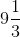Therefore the required third proportional is(iii) Rs 1.60, Rs 0.40

Let the required third proportional be x

Hence Rs 1.60, 0.40, x are in continued proportion

⇒ 1.60: 0.40 = 0.40: x

⇒ 1.60 × x = 0.40 × 0.40

⇒ x = (0.40 × 0.40) / 1.60

⇒ x = 0.1

Therefore required third proportional is 0.1

5. The ratio between 7 and 5 is same as the ratio between Rs x and Rs 20.50; find the value of x

Solution:

Given

Ratio between 7 and 5 is same as the ratio between Rs x and Rs 20.50

Hence, the value of x can be calculated as below

7: 5 = x: 20.50

5x = 7 × 20.50

x = (7 × 20.50) / 5

x = (143.5) / 5

x = 28.7

Therefore the value of x is 28.7

6. If (4x + 3y): (3x + 5y) = 6: 7, find:

(i) x: y

(ii) x, if y = 10

(iii) y, if x = 27

Solution:

(i) x: y

Given

(4x + 3y): (3x + 5y) = 6: 7

We can calculate x: y as below

7(4x + 3y) = 6(3x + 5y)

28x + 21y = 18x + 30y

28x – 18x = 30y – 21y

10x = 9y

x / y = 9 / 10

Therefore x: y is 9: 10

(ii) x, if y = 10

Given

(4x + 3y): (3x + 5y) = 6: 7

And y = 10

Hence we can calculate x as below

7(4x + 3 × 10) = 6(3x + 5 × 10)

7(4x + 30) = 6(3x + 50)

28x + 210 = 18x + 300

28x – 18x = 300 – 210

10x = 90

x = 90 / 10

x = 9

Therefore the value of x is 9

(iii) y, if x = 27

Given

(4x + 3y): (3x + 5y) = 6: 7

And x = 27

We can calculate x as below

7(4 × 27 + 3y) = 6(3 × 27 + 5y)

7(108 + 21y) = 6(81 + 5y)

756 + 21y = 486 + 30y

9y = 756 – 486

9y = 270

y = 270 / 9

y = 30

Therefore the value of y is 30

7. If (2y + 5x) / (3y – 5x) =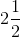, find:

(i) x: y

(ii) x, if y = 70

(iii) y, if x = 33

Solution:

(i) x: y

Given

(2y + 5x) / (3y – 5x) =Hence x: y can be calculated as below

(2y + 5x) / (3y – 5x) =We get

(2y + 5x) / (3y – 5x) = 5 / 2

2 [(2y + 5x)] = 5[(3y – 5x)]

4y + 10x = 15y – 25x

25x + 10x = 15y – 4y

35x = 11y

x / y = 11 / 35

x: y = 11: 35

Therefore x: y is 11: 35

(ii) x, if y = 70

Given

(2y + 5x) / (3y – 5x) =And y = 70

Hence x can be calculated as below

(2 × 70 + 5x) / (3 × 70 – 5x) =On calculating further, we get

(2 × 70 + 5x) / (3 × 70 – 5x) = 5 / 2

2 [140 + 5x] = 5 [210 – 5x]

280 + 10x = 1050 – 25x

25x + 10x = 1050 – 280

35x = 770

x = 770 / 35

x = 22

Therefore x is 22

(iii) y, if x = 33

Given

(2y + 5x) / (3y – 5x) =And x = 33

Hence y can be calculated as below

(2y + 5 ×33) / (3y – 5 × 33) =(2y + 5 ×33) / (3y – 5 × 33) = 5 / 2

2 [(2y + 165)] = 5 [(3y – 165)]

4y + 330 = 15y – 825

11y = 1155

y = 1155 / 11

y = 105

Therefore y is 105

Exercise 12(C)

1. Are the following numbers in proportion:

(i) 32, 40, 48 and 60?

(ii) 12, 15, 18 and 20?

Solution:

(i) 32, 40, 48 and 60

Given

Numbers are 32, 40, 48 and 60

If 32: 40 = 48: 60 then the ratios are in continued proportion

Hence we can find out as shown below

32: 40 = 48: 60

32 × 60 = 40 × 48

1920 = 1920

Since they are equal

Therefore they are in continued proportion

(ii) 12, 15, 18 and 20

Given

Numbers are 12, 15, 18 and 20

If 12: 15 = 18: 20 then the ratios are in continued proportion

Hence we can find out as shown below

12: 15 = 18: 20

12 × 20 = 15 × 18

240 = 270

240 ≠ 270

Since they are not equal

Therefore they are not in a continued proportion

2. Find the value of x in each of the following such that the given numbers are in proportion

(i) 14, 42, x and 75

(ii) 45, 135, 90 and x

Solution:

(i) 14, 42, x and 75

Given

Numbers are 14, 42, x and 75

If 14: 42 = x: 75 then they are in continued proportion

Hence we can find out as shown below

14: 42 = x: 75

14 × 75 = x × 42

x = (14 × 75) / 42

x = 1050 / 42

x = 25

Therefore the value of x is 25

(ii) 45, 135, 90 and x

Given

Numbers are 45, 135, 90 and x

If 45: 135 = 90: x then they are in continued proportion

Hence we can find out value of x as shown below

45: 135 = 90: x

45 × x = 90 × 135

x = (90 × 135) / 45

x = 12150 / 45

x = 270

Therefore the value of x is 270

3. The costs of two articles are in the ratio 7: 4. If the cost of the first article is Rs 2800; find the cost of the second article

Solution:

Given

Costs of two articles are in the ratio = 7: 4

Cost of first article = Rs 2800

Let us assume the cost of second article be x

Hence the value of second article can be calculated as shown below

7: 4 = 2800: x

7 / 4 = 2800 / x

7 × x = 2800 × 4

x = (2800 × 4) / 7

x = 11200 / 7

x = 1600

Therefore the cost of second article is Rs 1600

4. The ratio of the length and the width of a rectangular sheet of paper is 8: 5. If the width of the sheet is 17.5 cm; find the length

Solution:

Given

Ratio of the length and the width of a rectangular sheet of paper = 8: 5

Width of the sheet = 17.5 cm

Hence the value of length can be calculated as shown below

8: 5 = x: 17.5

8 / 5 = x / 17.5

5 × x = 8 × 17.5

x = (8 × 17.5) / 5

x = 140 / 5

x = 28

Therefore the length of sheet is 28 cm

5. The ages of A and B are in the ratio 6: 5. If A’s age is 18 years, find the age of B.

Solution:

Given

The ages of A and B are in the ratio = 6: 5

Age of A = 18 years

Let the age of B be x years

Hence the value of B’s age can be calculated as shown below

6: 5 = 18: x

6 / 5 = 18 / x

6 × x = 18 ×5

x = (18 × 5) / 6

x = 90 / 6

x = 15

Therefore the age of B is 15 years

6. A sum of Rs 10, 500 is divided among A, B and C in the ratio 5: 6: 4. Find the share of each.

Solution:

Given

Amount divided among A, B and C in the ratio = 5: 6: 4

Total amount divided = Rs 10500

Hence the sum of ratios can be calculated as below

Sum of ratios = 5 + 6 + 4

= 15

Hence the share’s of A, B and C can be calculated as below

A’s share = Rs (10500) / 15 × 5

= 700 × 5

= Rs 3500

B’s share = Rs (10500) / 15 × 6

= 700 × 6

=4200

C’s share = Rs (10500) / 15 × 4

= 700 × 4

= Rs 2800

Therefore the share of A, B and C are Rs 3500, Rs 4200 and Rs 2800

7. Do the ratios 15 cm to 2 m and 10 sec to 3 minutes form a proportion?

Solution:

Given

15 cm: 2 m:: 10 sec: 3 min

We know that,

1 m = 100 cm

1min = 60 sec

Hence 2 m = 200 cm

3 min = 180 sec

We can check that they form the proportion or not as shown below:

15: 200:: 10: 180

3: 40:: 1: 180

Therefore they do not form the proportion

8. Do the ratios 2 kg: 80 kg and 25 g: 625 g form a proportion?

Solution:

Given

2 kg: 80:: 25 g: 625 g

We can check that they form the proportion or not as shown below

2: 80:: 25: 625

1: 40:: 1: 25

Therefore they do not form the proportion

9. 10 kg sugar cost Rs 350. If x kg sugar of the same kind costs Rs 175, find the value of x

Solution:

Given

Cost of 10 kg sugar = Rs 350

Cost of x kg sugar = Rs 175

The value of x can be calculated as shown below

10 kg: x kg:: 350: 175

10 × 175 = 350 × x

350 x = 1750

x = 1750 / 350

x = 5

Therefore, 5 kg of sugar costs Rs 175

10. The length of two ropes are in the ratio 7: 5. Find the length of:

(i) shorter rope, if the longer one is 22.5 m

(ii) longer rope, if the shorter is 9.8 m

Solution:

(i) shorter rope, if the longer one is 22.5 m

Given

Ratio of length of two rope = 7: 5

And longer rope = 22.5 m

Let the shorter rope length be x

Hence, length of shorter rope can be calculated as below

7: 5 = 22.5: x

7x = 22.5 × 5

x = (22.5 × 5) / 7

x = 112.5 / 7

x = 16.07 m

Therefore the length of shorter rope is 16.07 m

(ii) longer rope, if the shorter is 9.8 m

Given

Ratio of length of two rope = 7: 5

And shorter rope = 9.8 m

Let the length of longer rope be x

Hence, the length of longer rope can be calculated as below

7: 5 = x: 9.8

5x = 9.8 × 7

x = (9.8 × 7) / 5

x = 68.6 / 5

x = 13.72 m

Therefore the length of longer rope is 13.72 m

11. If 4, x and 9 are in continued proportion, find the value of x

Solution:

Given

Numbers are 4, x and 9 are in continued proportion

Hence we can find out value of x as below

4: x = x: 9

x2 = 9 × 4

x = 36

x = 6

Therefore the value of x is 6

12. If 25, 35 and x are in continued proportion, find the value of x

Solution:

Given

Numbers are 25, 35 and x are in continued proportion

Hence we can find out value of x as below

25: 35 = 35: x

25 × x = 35 × 35

x = (35 × 35) / 25

x = 1225 / 25

x = 49

Therefore the value of x is 49